Example Questions

1 2 3 4 5 6 7 8 9 11 Next →

Example Question #101 : Linear / Rational / Variable Equations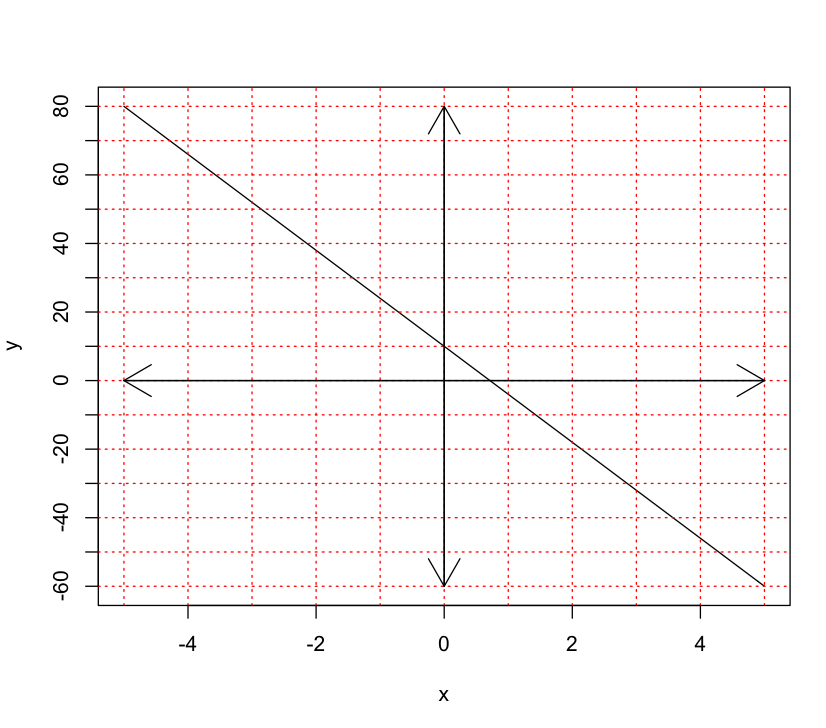Calculate the slope of the line.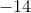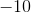Explanation:

In order to solve for the slope, we need to recall how to find the slope of a line.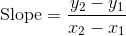, where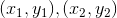are points on the line.

So we will pick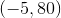, and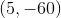.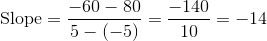Example Question #102 : Linear / Rational / Variable Equations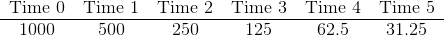What equation best represents the following table?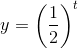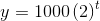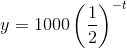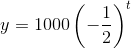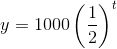Explanation:

The first step is to find the common ratio amongst the data.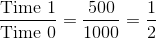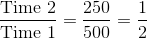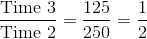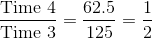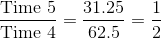We can that the common ratio is.

Now we need to set up an equation that will give an answer for any time.

We can set up an exponential decay model, the general equation is,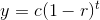, whereis the starting amount,is the common ratio andis time.

After plugging our numbers in, we get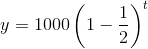Example Question #145 : Algebra

Sally sells custom picture frames.  Her monthly fixed costs are $350. It costs$10 to make each frame.  Sally sells her picture frames for \$35 each.

How many picture frames must Sally sell in order to break even?Explanation:

The break-even point is where the costs equal the revenues.

Let= # of frames sold

Costs: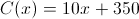Revenues: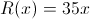Thus,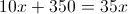So 14 picture frames must be sold each month to break-even.

Example Question #103 : Linear / Rational / Variable Equations

8 is 3 greater than the square root of an unknown number. What is the unknown number?Explanation:

In order to solve for the unknown number, you must write an equation and solve for a variable. 8 is 3 greater than the square root of an unknown number translates to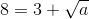To solve for a you must subtract 3 from both sides, and then square both sides.

As you can see,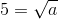and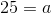So, the value of the unknown number is 25.

Example Question #43 : New Sat Math No Calculator

Solve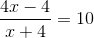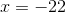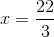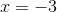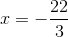Explanation:First multiply by the denominator on each side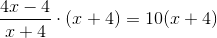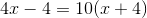Expand left side, and solve for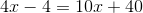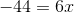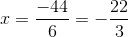1 2 3 4 5 6 7 8 9 11 Next →